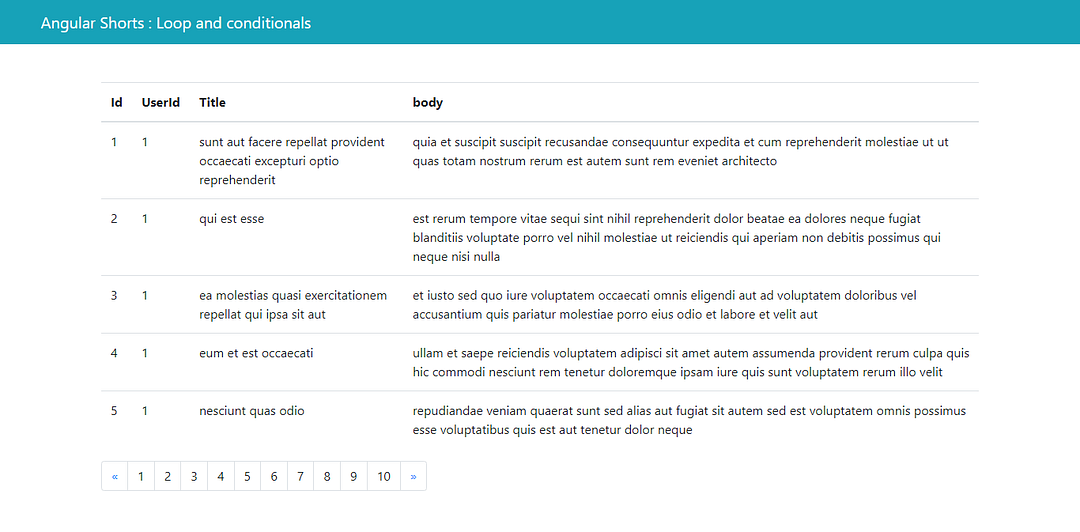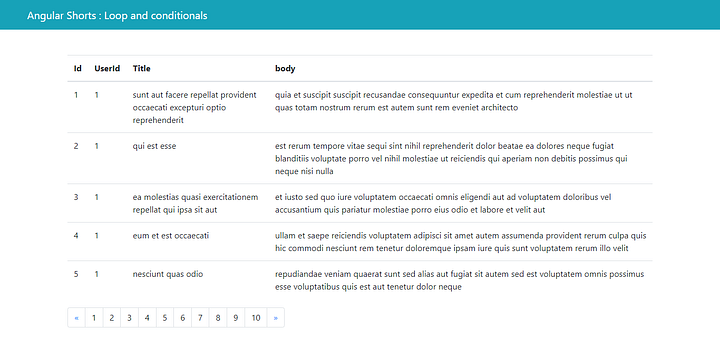# Angular Tutorials -Angular Pagination Without Any LibraryIn this short Angular tutorial ill show you how one can achieve pagination this is one of the angular interview questions asked in practical tests like data table in angular without using any other library .
So i am assuming that you already familiar on how to create a table in angular and populate data in it
so for pagination we will have the html code from the bootstrap as it is beautifully designed

``````<nav aria-label="Page navigation example">
<ul class="pagination">
<li class="page-item">
<a class="page-link" href="#" aria-label="Previous">
<span aria-hidden="true">&laquo;</span>
</a>
</li>
<li class="page-item"><a class="page-link" href="#">1</a></li>
<li class="page-item"><a class="page-link" href="#">2</a></li>
<li class="page-item"><a class="page-link" href="#">3</a></li>
<li class="page-item">
<a class="page-link" href="#" aria-label="Next">
<span aria-hidden="true">&raquo;</span>
</a>
</li>
</ul>
</nav>``````now the li with class name ‘page-item ’ are the actual pages so
how many pages we need that is basically the length of the data lets assume its 100 divided by no of records we need in one page so it came 10 pages
now 10 pages is the number and ngfor does not work with numbers it works with arrays so we need an array of length 10 so we will have a function which will return an array of the length passed as parameter init

``````getArrayFromNumber(number){
return new Array(number/10);
}``````

now we will have an array and we can create pages from it like

``````<nav aria-label="Page navigation example">
<ul class="pagination">
<li class="page-item">
<a class="page-link" href="#" aria-label="Previous">
<span aria-hidden="true">&laquo;</span>
</a>
</li>
<li class="page-item"
*ngFor="let pageNumber of getArrayFromNumber            (data.length);let i=index">
<a class="page-link" href="#">1</a></li>

<li class="page-item">
<a class="page-link" href="#" aria-label="Next">
<span aria-hidden="true">&raquo;</span>
</a>
</li>
</ul>
</nav>``````

we are using index as ‘getArrayFromNumber()’ function returns empty array of specific length now the main part
in angular thier is a pipe that is slice pipe which is same as normal slice funtion it takes two parameters first is start Index and the other is end index
and it will give us records stating from startindex till (end index -1)
so let say if i want top 10 records from an array in ngfor i ll use it like

``````<tr *ngFor="let item of data | slice :0:10">
</tr>``````

now you have the secret sauce you can do it n n number of ways how i used it was created two properties in my component startIndex and endIndex
and updating them on page change
you can see the video over here which is in more depth